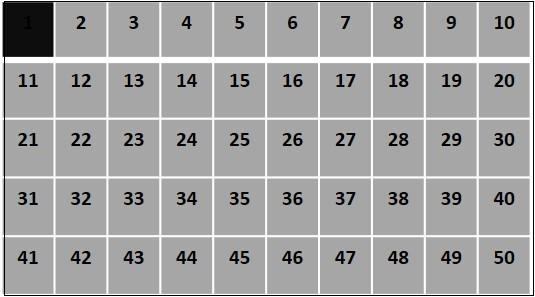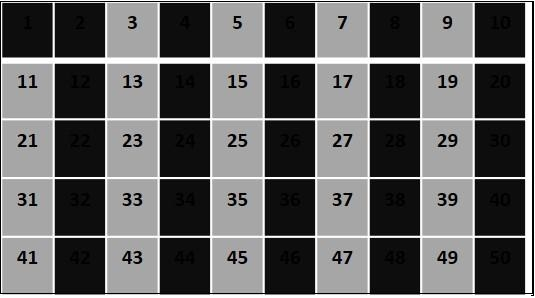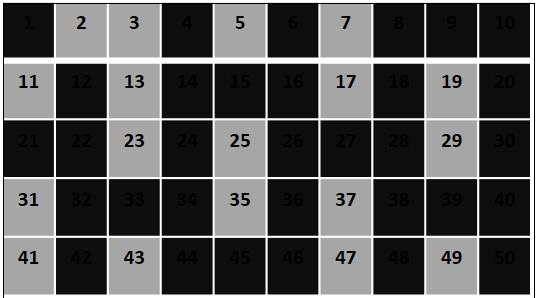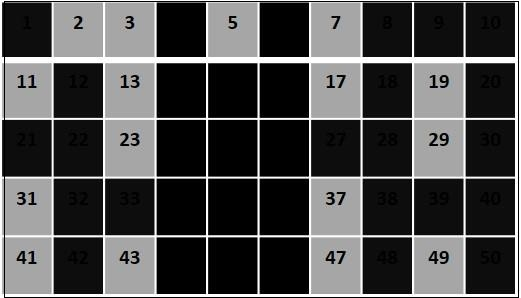# Sieve of Eratosthenes in java

Sieve of Eratosthenes is the ancient algorithm to find prime numbers up to a given number.

#### Algorithm

1. Generate integers from 2 to n (Given number).2. Counting from 2 mark every 2nd integer. (multiples of 2)3. Now, starting from 3 mark every third integer. (multiples of 3)4. Finally, marking from 5 mark every 5th integer.(multiples of 5)## Program

import java.util.Scanner;

public class SievePrimeFactors  {
public static void main(String args[]) {
Scanner sc = new Scanner(System.in);
System.out.println("Enter a number");
int num = sc.nextInt();
boolean[] bool = new boolean[num];

for (int i = 0; i< bool.length; i++) {
bool[i] = true;
}
for (int i = 2; i< Math.sqrt(num); i++) {
if(bool[i] == true) {
for(int j = (i*i); j<num; j = j+i) {
bool[j] = false;
}
}
}
System.out.println("List of prime numbers upto given number are : ");
for (int i = 2; i< bool.length; i++) {
if(bool[i]==true) {
System.out.println(i);
}
}
}
}

## Output

Enter a number
50
List of prime numbers up to given number are :
2
3
5
7
11
13
17
19
23
29
31
37
41
43
47